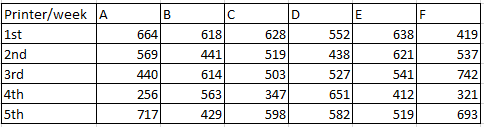### SBI PO 2017 Question 13

Instructions

Study the following table carefully and answer the questions.Question 13

# Which of the following printer printed maximum number of pages in all the given weeks together?

Solution

Total pages printed by A = 2646

Total pages printed by B = 2665

Total pages printed by C = 2595

Total pages printed by D = 2750

Total pages printed by E = 2731

Total pages printed by F = 2712

Hence, Maximum pages printed by printer D only.